# 2nd PUC Maths Previous Year Question Paper March 2019

Students can Download 2nd PUC Maths Previous Year Question Paper March 2019, Karnataka 2nd PUC Maths Model Question Papers with Answers helps you to revise the complete Karnataka State Board Syllabus and score more marks in your examinations.

## Karnataka 2nd PUC Maths Previous Year Question Paper March 2019

Time: 3 Hrs 15 Min
Max. Marks: 100

Part-A

Answer ALL the following questions: (10 × 1 = 10)

Question 1.
Define Binary Operation.
A binary operation * on a set ‘A’ is defined as a function.
* : A × A → A
a * b = ab + 1 ∈ Z ∀ a, b, ∈ Z
∴ * is binary operation on Z.

Question 2.
Find the principal value of cos-1$$\left(-\frac{1}{2}\right)$$.
$$\frac{2 \pi}{3}$$

Question 3.
Define a Scalar Matrix.
In a square matrix the principal diagonal elements are same and all the other elements are zeroes.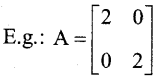Question 4.
Find a value of x for which3 – x2 = 3 – 8 ⇒ x2 = 8 ⇒ x = ±2$$\sqrt{2}$$

Question 5.
If y = sin(x2 + 5) then find $$\frac{d y}{d x}$$
y = sin(x2 + 5)
$$\frac{d y}{d x}$$ = cos(x2 + 5)(2x)

Question 6.
Find $$\int(1-x) \sqrt{x} d x$$
$$\int(1-x) \sqrt{x} d x$$Question 7.
Find a value of x for which x(î + ĵ + k̂) is a unit vector.
x(î + ĵ + k̂) = 1 ⇒ xî+ xĵ+ xk̂ = 1
⇒$$\sqrt{x^{2}+x^{2}+x^{2}}$$ = 1 ⇒ $$\sqrt{3 x^{2}}$$ = 1 ⇒ x = $$\frac{1}{\sqrt{3}}$$

Question 8.
If a line has direction ratios 2, -1, -2 then determine its direction cosines.
$$\left(\frac{2}{3}, \frac{-1}{3}, \frac{-2}{3}\right)$$

Question 9.
Define objective function in Linear Programming Problem.
The linear function Z = ax + by, where ‘a’ and ‘b’ are constants which has to be maximised or minimised is called objective function.

Question 10.
If P(E) = 0.6, P(F) = 0.3 and P(E n f) = 0.2 then find P(F/E).
$$P(F / E)=\frac{P(F \cap E)}{P(E)}=\frac{0.2}{0.6}=\frac{2}{6}=\frac{1}{3}$$Part-B

Answer any TEN questions: (10 × 2 = 20)

Question 11.
Show that the function f: N → N given by f(x) = 2x is one-one but not onto.
The function/is one-one, for f(X1) = f(x2)
⇒ 2x1 = 2x2 ⇒ x1 = x2 Further,/is not onto, as for 1 ∈ N, there does not exist any x in N such that f(x) = 2x = 1.

Question 12.
Prove that sin-1 x + cos-1 x = $$\frac{\pi}{2}$$, x ∈ [-1,1].
Let sin-1 x = θ then.
x = sin θ ⇒ x = cos($$\frac{\pi}{2}$$ – θ)
⇒ Cos-1x = $$\frac{\pi}{2}$$ – θ ⇒ θ + cos-1 x = $$\frac{\pi}{2}$$
∴ sin-1 x + cos-1 x = $$\frac{\pi}{2}$$

Question 13.
Write cot-1$$\left(\frac{1}{\sqrt{x^{2}-1}}\right)$$, x > 1 simplest form.
Let x = sec q ⇒ q = sec-1 x.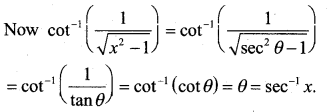Question 14.
Find the area of the triangle with vertices (2, 7), (1, 1) and (10, 8) using determinant method.
The area of triangle is given by= $$\frac { 1 }{ 2 }$$ |[-2 (1 – 8) -7 (1 – 10) + 1 (8 – 10)]|
= $$\frac { 1 }{ 2 }$$ |-14 + 63 – 2| = $$\frac { 47 }{ 2 }$$ sq.units

Question 15.
Find $$\frac{d y}{d x}$$ if y = (logx)cosx.
Let y = (log x)cosx
⇒ log y = cos x log (logx)
⇒ $$\frac{d}{d x}$$ log y = $$\frac{d}{d x}$$ cos x log(log x)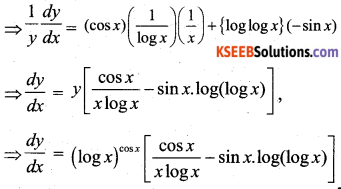Question 16.
Find ax + by2 = cosy then find $$\frac{dy}{d x}$$
ax + by2 = cosy
⇒ $$\frac{d}{d x}$$ax + $$\frac{d}{d x}$$by2 = $$\frac{d}{d x}$$cosy
⇒ a + 2by $$\frac{dy}{d x}$$ = (-sin y)$$\frac{dy}{d x}$$ dx dx
(2by + sin y) $$\frac{dy}{d x}$$ = -a ⇒ $$\frac{d}{d x}$$ = $$-\frac{a}{2 b y+\sin y}$$

Question 17.
Find the approximate change in the volume V of a cube of side x meters caused by increasing side by 2%.
Note that V = x3
or dV = $$\left(\frac{d V}{d x}\right)$$ ∆x = (3x2) ∆x
= (3x2) (0.02x) = 0.06x3m3
Thus, the approximate change in volume is 0.06 x3m3Question 18.
Find $$\int \frac{1}{\cos ^{2} x(1-\tan x)^{2}} d x.$$
Put (1 – tan x) = t ⇒ – sec2 x dx = dtQuestion 19.
Find $$\int \sin 2 x \cos 3 x d x$$
W.K.T sin x cosy = $$\frac { 1 }{ 2 }$$[sin(x + y) +sin(x – y)]
Then $$\int \sin 2 x \cos 3 x d x=\frac{1}{2}\left[\int \sin 5 x d x-\int \sin x d x\right]$$
= $$\frac { 1 }{ 2 }$$ [$$\frac { 1 }{ 5 }$$cos 5x + cos x] + C = $$\frac { -1 }{ 10 }$$ cos5x + $$\frac { 1 }{ 2 }$$ cos x + C.

Question 20.
Find the order and degree (if defined) of the differential equation
$$\left(\frac{d^{3} y}{d x^{2}}\right)^{2}+\cos \left(\frac{d y}{d x}\right)$$=0
Order = 2, Degree = not defined.

Question 21.
If $$(\vec{a}+\vec{b}) \cdot(\vec{a}-\vec{b})$$ = 8 and $$|\vec{a}|$$ = 8$$(\vec{b})$$ then find $$|\vec{b}|$$.Question 22.
Find the projection of the vector ā = 2î + 3ĵ + 2k̂ and b̄ = î – ĵ + k̂
Projection of vector ā on b̄ is given by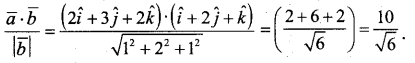Question 23.
Find the distance of the point (3, -2, 1) from the plane 2x – y + 2Z + 3 = 0.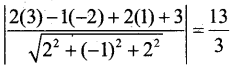Question 24.
Probability of solving specific problem independently by A and B are $$\frac { 1 }{ 2 }$$ and $$\frac { 1 }{ 3 }$$ respectively. If both try to solve the problem independently, find the probability that the problem is solved.
Given P(A) = $$\frac { 1 }{ 2 }$$ and P(B) = $$\frac { 1 }{ 23}$$
(i) The problem is solved
= 1 – P(not solved) = 1 – P(A’ ∩ B’)
= 1 – P(A’) P(B’) = 1 – [(1 – $$\frac { 1 }{ 2 }$$ – (1 – $$\frac { 1 }{ 3 }$$]
= 1 – ($$\frac { 1 }{ 2 }$$ × $$\frac { 2 }{ 3 }$$) = 1 – $$\frac { 1 }{ 3 }$$ = $$\frac { 2 }{ 3 }$$

(ii) Exactly one of them solves problem
= P(A ∩ B) + P(A ∩ B’)
= P(A’) + P(B) + P(A) P(B’)
$$=\left(1-\frac{1}{2}\right)\left(\frac{1}{3}\right)+\frac{1}{2}\left(1-\frac{1}{3}\right)$$
$$=\left(\frac{1}{2}\right)\left(\frac{1}{3}\right)+\frac{1}{2}\left(\frac{2}{3}\right)=\frac{1}{2}$$

Part-C

Answer any TEN questions: (10 × 3 = 30)

Question 25.
Check whether the relation R in 1R of real numbers defined by R = {(a, b): a < b3} is reflexive, symmetric or transitive.
(i) For (a, a) ∈R, a < a3 which is false.
As for a = -2, a3 = -8
∴ a $$\nleq$$ a3 as – 2 $$\nleq$$ – 8
∴ R is not reflexive.

(ii) For (a, b), a < b3 and (b, a), b < a3 which is false
As for a = 1 and b = 2
a < b3 as 1 < 8
Now, b $$\nleq$$ a3 as 2 $$\nleq$$ 1
∴ R is not symmetric.

(iii) For (a, b), (b, c) and (a, c)
a < b3 and b < c3
Now a = 25, b = 3, c = 2
a < b3 and 25 < 27
b < c3 and 3 < 8
but a $$\nleq$$ c3 as 25 $$\nleq$$ 8
.’. R is not transitive.
Hence R is neither reflexive, nor symmetric and nor transitive.

Question 26.
Prove that
cos-1 ($$\frac { 4 }{ 5 }$$) + cos-1 ($$\frac { 12 }{ 13 }$$) = cos-1 ($$\frac { 33 }{ 65 }$$)
Let
cos-1 ($$\frac { 4 }{ 5 }$$) ⇒ cos A = $$\frac { 4 }{ 5 }$$ ⇒ Sin A = $$\sqrt{1-\frac{16}{25}}=\frac{3}{5}$$
cos-1 ($$\frac { 12 }{ 13 }$$) = cos B = $$\frac { 12 }{ 13 }$$ ⇒ sin B = $$\sqrt{1-\frac{144}{169}}=\frac{5}{13}$$
cos(A + B) = cosA.cosB – sinA.sinB $$=\frac{4}{5} \cdot \frac{12}{13}-\frac{3}{5} \cdot \frac{5}{13}=\frac{48}{65}-\frac{15}{65}=\frac{33}{65}$$
cos(A + B) = $$\frac { 33 }{ 65 }$$ ⇒ A + B = cos-1 ($$\frac { 33 }{ 65 }$$)
cos-1 ($$\frac { 4 }{ 5 }$$) + cos-1 ($$\frac { 12 }{ 13 }$$) = cos-1 ($$\frac { 33 }{ 65 }$$)Question 27.
By using elementary transformations, find the inverse of the matrix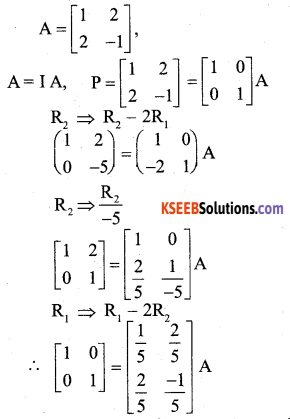Question 28.
If x = a(θ + sin θ), y = a(1 – cosθ) then show that $$\frac{d y}{d x}=\tan \left(\frac{\theta}{2}\right)$$
We have
$$\frac{d x}{d \theta}$$ = a(1 + cos θ), $$\frac{d y}{d \theta}$$ = a(sin θ)Question 29.
Verify Rolle’s theorem for the function f(x) = x2 + 2x – 8, x ∈ [-4, 2].
f(x) is a polynomial in x. Hence it is continuous over [-4, 2] and differentiable over (-4,2).
f(-4) = (-4)2 + 2 (-4) – 8 = 16 – 8 – 8 = 0
f(2) = 4 + 4 – 8 = 0
∴ f(-4) = f(2)
∴ All the conditions of the Rolle’s theorem are satisfied.
∴ there exists a c ∈[-4,2] such that
f'(c) = 0 f'(x) = 2x + 2
f(c) = 2c + 2
f(c) = 0
⇒ 2c + 2 = 0
⇒ 2c = -2
⇒ c = -1 ∈[-4, 2]
∴ Rolle’s theorem is verified.

Question 30.
Find the intervals in which the function f given by f(x) = 2X3 – 3x2 – 36x + 7 is strictly increasing.
f(x) = 2x3 – 3x2 – 36x + 7
f ‘(x) = 6x2 – 6x – 36
f ‘(x) = 0 ⇒ 6x2 – 6x – 36 = 0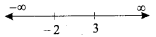⇒ 6(x2 – x – 6) = 0
⇒ 6(x – 3)(x + 2) = 0
6 ≠ 0, x = 3, x = -2Question 31.
Find $$\int x \log x d x$$Question 32.
Evaluate $$\int_{0}^{\frac{\pi}{2}} \frac{\sin x}{1+\cos ^{2} x} d x$$Question 33.
Find the area of the region bounded by the curve y2 = 4x and the line x = 3.
Given curve is y2 = 4x and line is x = 3
y2 = 4x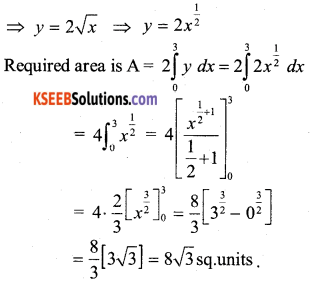Question 34.
Form the differential equation of the family of curves y = ae3x + be-2x by eliminating arbitrary constants a and b.
Given, y = a e3x + b e-2x …. (1)
y’ = (a e3x) 3 – (be-2x) 2
y’ = 3 a e3x – 2 be-2x …. (2)
From (1) and (2)
y = a e3x + b e-2x × 3
y’ = 3a e3x – 2b e-2x × 1Diff
⇒ 3y’ – y” = 5be-2x
3y’ – y” = -10be-2x ….(4)
From (3) & (4)
3y’ – y” = (-2) (5be-2x)
3y’ – y” = -2[3y – y’]
3y’ – y” = -6y + 2y’
y” – y’ – 6y = 0.Question 35.
Find a unit vector perpendicular to each of the vector $$(\vec{a}+\vec{b})$$ and $$(\vec{a}-\vec{b})$$, where ā = î + ĵ + k̂, b̄ = î + 2ĵ + 3k̂.Question 36.
Show that the four points with position vectors: 4î + 8ĵ + 12k̂, 2î + 4ĵ + 6k̂, 3î + 5ĵ + 4k̂ and 5î + 8ĵ + 5k̂ are coplanar.
Let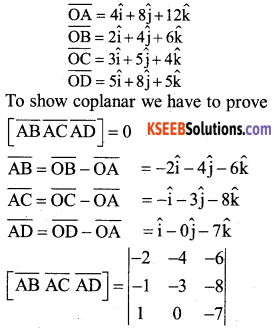= -2(21 + 0) + 4(7 + 8) – 6(0 + 3)
= -42 + 60 – 18 = -60 + 60 = 0
∴ A, B, C, D are coplanar.

Question 37.
Find the vector equation of the plane passing through the points R(2, 5,-3), S(-2, -3, 5) and T(5, 3, -3).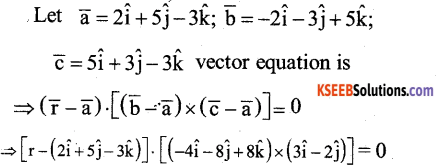Theorem: Derive intercept form of the equation of a plane
Let the equation of a plane be
Ax + By + Cz + 0 = 0 (1)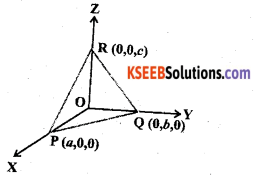Let the plane cuts X – axis at p(a, 0, 0), Y-axis at Q(0, b, 0), Z-axis at R(0, 0, c) substituting P(a, 0, 0) in equation (1)
Aa + D = 0 ⇒ Aa = -D ⇒ A = $$\frac{-D}{a}$$
substituting Q(0, b, 0) in equation (1)
Bb + D = 0 ⇒ Bb = D ⇒ B = $$\frac{-D}{b}$$
R(0, 0, c) in equation (1)
Cc + D = 0 ⇒ Cc = -D ⇒ C = $$\frac{-D}{c}$$
substituting A, B, C in (1)
$$\left(\frac{-D}{a}\right) x+\left(\frac{-D}{b}\right) y+\left(\frac{-D}{c}\right) z=-D$$
Dividing through out by -D
$$\Rightarrow \frac{x}{a}+\frac{y}{b}+\frac{z}{c}=1$$
is the required equation of the plane in intercept formQuestion 38.
An insurance company insured 2000 scooter drivers, 4000 car drivers and, 6000 truck drivers. The probability of an accident is 0.01, 0.03 and 0.15 respectively. One of the insured persons meets with an accident. What is the probability that he is a scooter driver?
Let S : be Insured person is scooter driver
C : be Insured person is car driver
T : be Insured person is truck driver
Let ‘A’ be the event “meets with an accident”.Part-D

Answer any SIX questions: (6 × 5 = 30)

Question 39.
Let f: N → Y be a function defined as
f(x) = 4x + 3, where
y = {y ∈ N: y = 4x + 3 for some x ∈ N}. Show that f is invertible. Find the inverse of f.
f:N → Y
f(x) = 4x + 3
To prove that / is one – one
Let f(x1) = f(x2)
4x1 + 3 = 4x2 + 3
4x1 = 4x2
∴ x1 = x2.
∴ f is one – one
To prove that f is onto
Let y ∈ Y there exits x ∈ N such that
f(x) = y
4x + 3 = y
4x = y – 3, x = $$\frac{y-3}{4}$$
∴ f is one-one and onto
⇒ f is bijective. Hence f is invertible
∴ f-1(y) = $$\frac{y-3}{4}$$

Question 40.
If A = $$\left[ \begin{matrix} 1 & 2 & 3 \\ 3 & -2 & 1 \\ 4 & 2 & 1 \end{matrix} \right]$$ then show that A2 – 23A – 40I = 0
We haveQuestion 41.
Solve the following system of linear equations by matrix method:
3x – 2y + 3z = 8
2x + y – z = 1
4x – 3y + 2z = 4
The system of equations can be written in the form A X = B where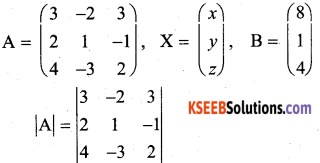|A| = 3(2 – 3) + 2(4 + 4) + 3(-6 -4) = -17
Hence, A is non singular matrix and so its inverse exists. Now
A11 = -1 A12 = -8 A13 = -10
A21 = -5 A22 = -6 A23 = +1
A31 = -1 A32 = +9 A33 = +7Question 42.
If y = (sin-1 x). show that
$$\left(1-x^{2}\right) \frac{d^{2} y}{d x^{2}}-x\left(\frac{d y}{d x}\right)=0$$
Given that y = sin-1 x, we have
y1 = $$\frac{1}{\sqrt{1-x^{2}}}$$ i.e., (1 – x2)y12 = 1
so (1 – x2). 2y1y2 + y12(0 – 2x) = 0
Hence (1 – x2) y2 – xy1 = 0

Question 43.
The length x of a rectangle is decreasing at the rate of 3 cm / min and the width y is increasing at the rate of 2 cm / min. When x = 10 cm and y = 6 cm, find the rates of change of
(i) the perimeter and
(ii) the area of the rectangle.
Since the length x is decreasing and the width y is increasing with respect to time, we have
$$\frac{d x}{d t}$$ = – 3 cm /min and $$\frac{d y}{d t}$$ = 2 cm/mm
(a) The perimeter P of a rectangel is given by P = 2 (x + y)
Therefore $$\frac{d P}{d t}$$ = $$2\left(\frac{d x}{d t}+\frac{d y}{d t}\right)$$ = 2 (-3 + 2)
= – 2 cm / min

(b) The area A of the rectangle is given A = x.y
Therefore $$\frac{d A}{d t}$$ = $$\frac{d x}{d t}$$ . y + x. $$\frac{d y}{d t}$$
= -3(6)+ 10(2) (as x= 10 cm and y = 6 cm)
= 2 cm2/min.Question 44.
Find the integral of $$\frac{1}{x^{2}-a^{2}}$$ with respect to x and hence evalauate $$\int \frac{1}{x^{2}-16} d x$$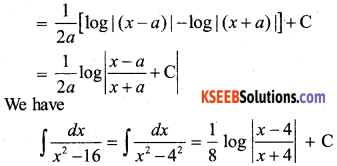Question 45.
Using the method of integration, find the smaller area enclosed by the circle x2 + y2 = 4 and the line x + y = 2.
Given circle x2 + y2 = 4 and the line is x + y = 2
x2 + (2 – x)2 = 4
[∴ y = 2 – x]2x2 – 4x = 0 ⇒ 2x(x – 2) = 0 ⇒ x = 0, 2
Now, x2 + y2 = 4 and x + y = 2
⇒ y2 = 4 – x2 y = 2 – x,
⇒ y = $$y=\sqrt{2^{2}-x^{2}}$$
Required Area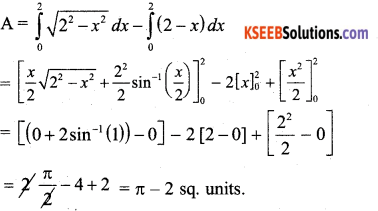Question 46.
Find the general solution of the differential equation $$\frac{d y}{d x}$$+ (secx)y = tanx, ( 0 ≤ x ≤ $$\frac{\pi}{2}$$).
Given circle x2 + y2 = 4 and the line is x + y = 2
x2 + (2 – x)2 = 4 [∴ y = 2 – x]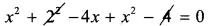2x2 – 4x = 0 ⇒ 2x(x – 2) = 0 ⇒ x = 0, 2
Now, x2 + y2 = 4 and x + y = 2
⇒ y2 = 4 – x y = 2 – x,
⇒ y = $$\sqrt{2^{2}-x^{2}}$$
Required AreaQuestion 47.
Derive the equation of a line in space passing through a given point and parallel to a given vector in both vector and Cartesian form.Let $$\overrightarrow{\mathrm{r}}$$ be any position vector on the line
‘L’ and $$\overrightarrow{\mathrm{a}}$$ given point on L.
clearly, $$\overrightarrow{\mathrm{AP}}$$ || $$\overrightarrow{\mathrm{b}}$$
$$\text { ie, } \overrightarrow{\mathrm{AP}}=\lambda \overrightarrow{\mathrm{b}}$$
where λ – some Real number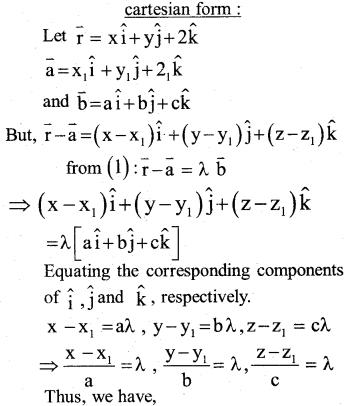Question 48.
Five cards are drawn successively with replacement from a we!! shuffled deck of 52 cards. What is the probability that
(i) all the five cards are spades?
(ii) only five three cards are spaces?Part-E

Answer any ONE question: (1 × 10 = 10)

Question 49.
(a) Prove that $$\int_{0}^{a} f(x) d x=\int_{0}^{a} f(a-x) d x$$ and hence evaluate $$\int_{0}^{\frac{\pi}{2}} \frac{\cos ^{5} x}{\sin ^{5} x+\cos ^{5} x} d x$$
Proof: a – x = t
– dx = dt
dx = -dt
L.L: when x = 0, t = a
U.L: When x = a, t = 0(b) Evaluate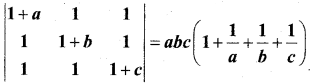Taking a, b, c commn from R1, R2, R3Question 50.
Minimise and Maximise
z = 5x + 10y
Subject to the constraints :
x + 2y ≤ 120
x + y ≥ 60
x – 2y ≥ 0
x ≥ 0 and y ≥ 0.
Draw the graph of the line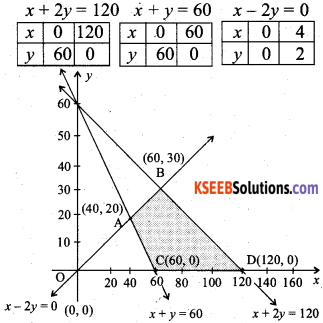Put (0, 0) in the inequality x + 2y ≤ 120
0 + 2(0) ≤ 120
0 ≤ 120 (True)
∴ The half plane lies towards the origin
P (0, 0) in the inequality x + y ≥ 0
0 + 0 ≥ 0 (False)
∴ The half plane lies away from the origin
Put (0, 1) in the inequality x – 2y ≥ 0
0 – 2(1) ≥ 0
-2 ≥ 0 (False)
∴ The half plane does not contain (0, 1)
Check the point (1, 0) in the inequality
x – 2y ≥ 0
1 – 2 (0) ≥ 0
⇒ 1 ≥ 0 (True)
∴ The half plane contains (1,0)
So the half plane is towards x – axis
Corner points Max & Min z = 5x+ 10y
A (40,20) z = 400
B (60, 30) z = 600 → Maximum
C (60, 0) z = 300 → Minimum
D (120, 0) z = 600 → Maximum
∴ At C (60, 0) z is minimum
At B (60,30), D (120,0) z is maximum

(b) Find the value of K, if
f(x) = $$\left\{\begin{array}{ll} {K x+1,} & {\text { if } x \leq 5} \\ {3 x-5} & {\text { if } x>3} \end{array}\right.$$
is continuous at x = 5. (4)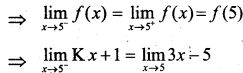⇒ K = $$\frac { 9 }{ 5 }$$